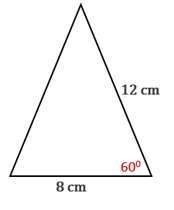Chapter 11.CT, Problem 15CT### Elementary Geometry for College St...

6th Edition
Daniel C. Alexander + 1 other
ISBN: 9781285195698

#### Solutions

Chapter
Section### Elementary Geometry for College St...

6th Edition
Daniel C. Alexander + 1 other
ISBN: 9781285195698
Textbook Problem
3 views

# Use one of the three forms for area (such as the form A = 1 2 b c sin ⁡ α ) to find the area of the triangle shown. Answer to the nearest whole number.___________To determine

To find:

The area of the triangle using the formula A=12bcsinα to the nearest whole number.

Explanation

Given:

The triangle shown below.

Approach:

Formula to find the area of a triangle is A=12bcsinα.

Calculation:

From the given diagram,

Let b=8 cm, c=12 cm, and the angle included between b and c be α=600.

To find the area of the triangle, substitute b=8 cm, c=12 cm, and α=600 in the formula

A

### Still sussing out bartleby?

Check out a sample textbook solution.

See a sample solution

#### The Solution to Your Study Problems

Bartleby provides explanations to thousands of textbook problems written by our experts, many with advanced degrees!

Get Started